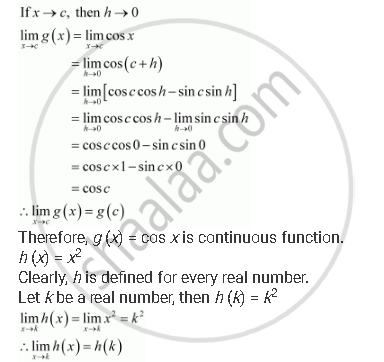Share

# Show that the Function Defined by F (X) = Cos (X2) is a Continuous Function. - CBSE (Commerce) Class 12 - Mathematics

#### Question

Show that the function defined by f (x) = cos (x2) is a continuous function.

#### Solution

The given function is (x) = cos (x2)

This function f is defined for every real number and f can be written as the composition of two functions as,

f = g o h, where g (x) = cos x and h (x) = x2

[∴(goh)(x) = g(h(x)) = g(x^2) = cos(x^2) = f(x)]

It has to be first proved that (x) = cos x and h (x) = x2 are continuous functions.

It is evident that g is defined for every real number.

Let c be a real number.

Then, g (c) = cos c

Put x = c + hTherefore, h is a continuous function.

It is known that for real valued functions and h,such that (h) is defined at c, if is continuous at and if is continuous at (c), then (g) is continuous at c.

Therefore, f(x) = (goh)(x) = cos(x^2) is a continuous function.

Is there an error in this question or solution?

#### APPEARS IN

NCERT Solution for Mathematics Textbook for Class 12 (2018 to Current)
Chapter 5: Continuity and Differentiability
Q: 31 | Page no. 161

#### Video TutorialsVIEW ALL 

Solution Show that the Function Defined by F (X) = Cos (X2) is a Continuous Function. Concept: Algebra of Continuous Functions.
S